Video Tutorial Atom Economy

Quick Notes Atom Economy

• Percentage atom economy is a way of showing how many of the reacting particles end up in the desired product for a particular reaction, shown as a percentage.
• calculated using: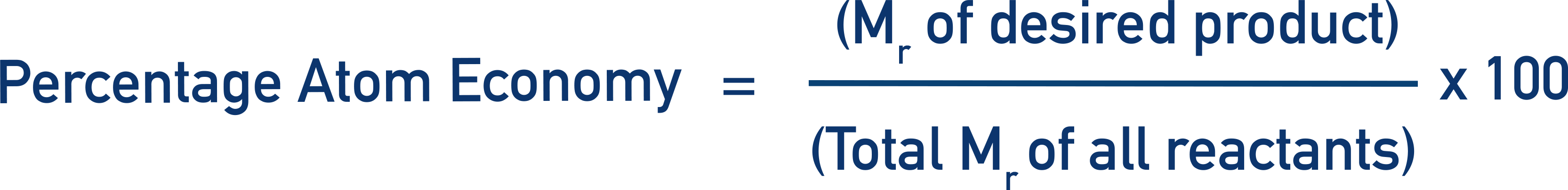• Values are theoretical and have no link to experimental measurements.
• Reactions in which only one product is produced will always have a percentage atom economy of 100%.

Full Notes Atom Economy (how to calculate)

Percentage atom economy is a way of showing, as a percentage, how much of the reactants for a particular reaction end up in the desired product.

Very often, a reaction won’t just produce a desired product, other ‘waste’ products will also be formed. By combining the relative masses (Mr) of all reactants and comparing them to the relative mass of the desired product, we can find out how much of the reactants ‘end up’ in the desired product. To make things easier, we express the comparison as a percentage. It is calculated using the formula: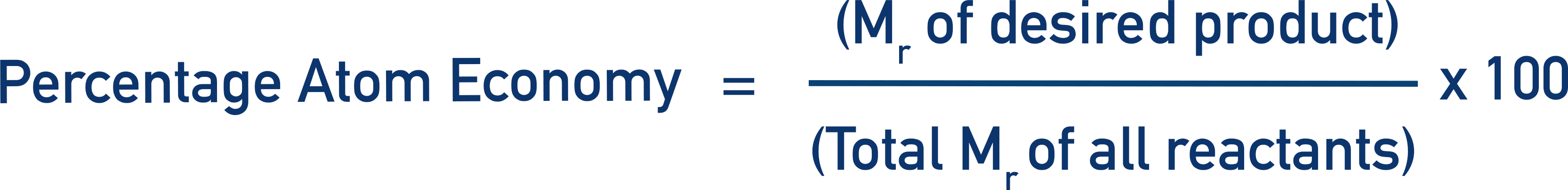Note - Sometimes you will see this equation as being the (relative mass of desired product) divided by (total relative masses of products). This is the same as the above equation as conservation of mass means the total relative masses of reactants will always be the same as the total relative masses of products. It makes no mathematical difference which way it is written.

For a general reaction, A + B, react together to produce C + D, if C is the desired product, then: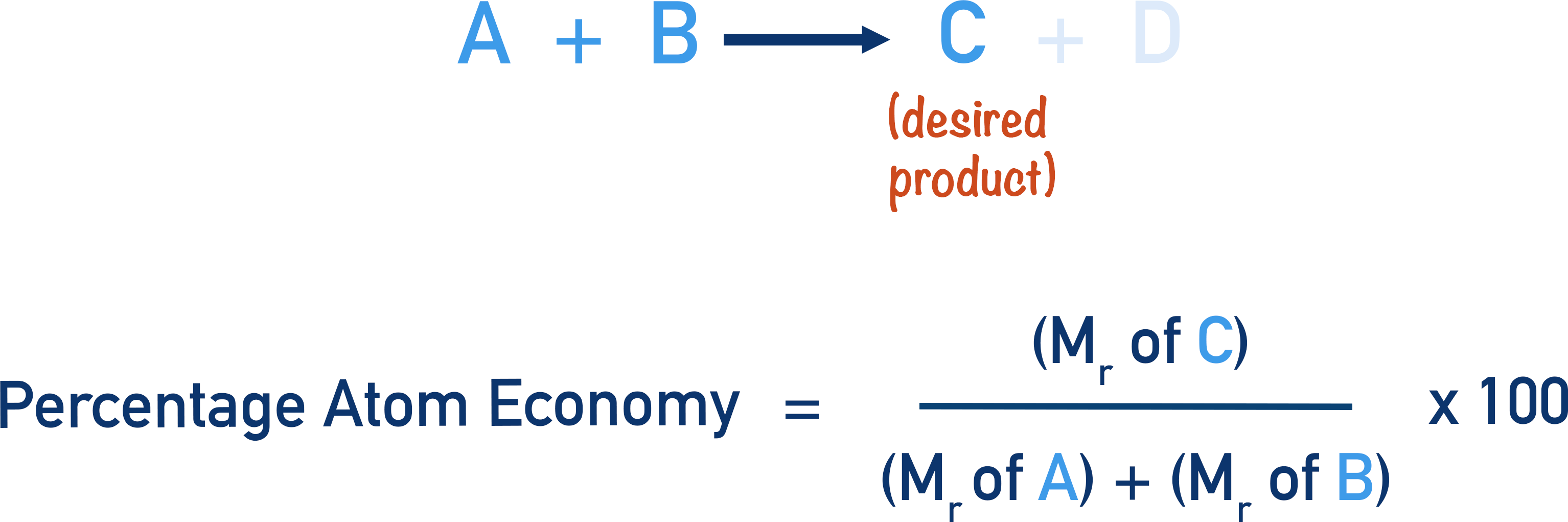If, as can sometimes happen, a reaction produces only one product, its atom economy will always be 100% as all the atoms of the reactants end up in the only product! For example, if A + B react together and only produce C, then: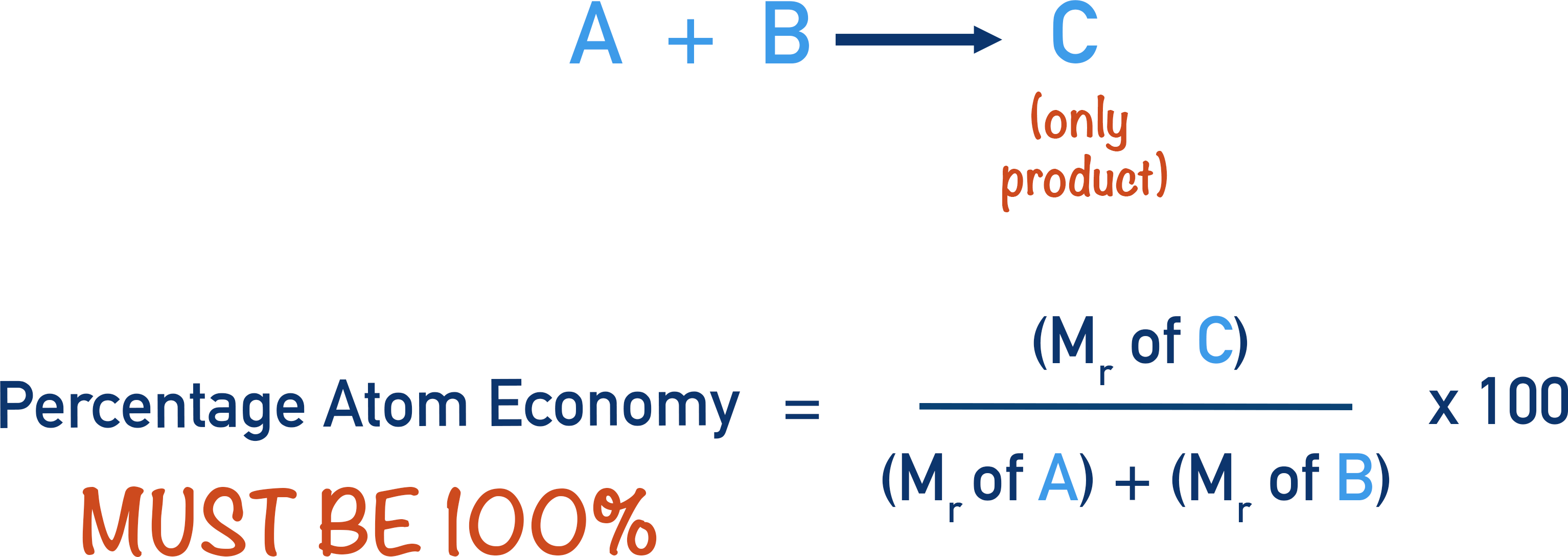High atom economy reactions are a good thing as less reactant mass is ‘wasted’ in forming products that aren’t wanted. Be careful though – just because a reaction has a high percentage atom economy doesn’t mean it has a high yield of product (see below).

## Examples

Find the percentage atom economy for the production of Iron (Fe) from the reaction between Iron (III) Oxide and Carbon.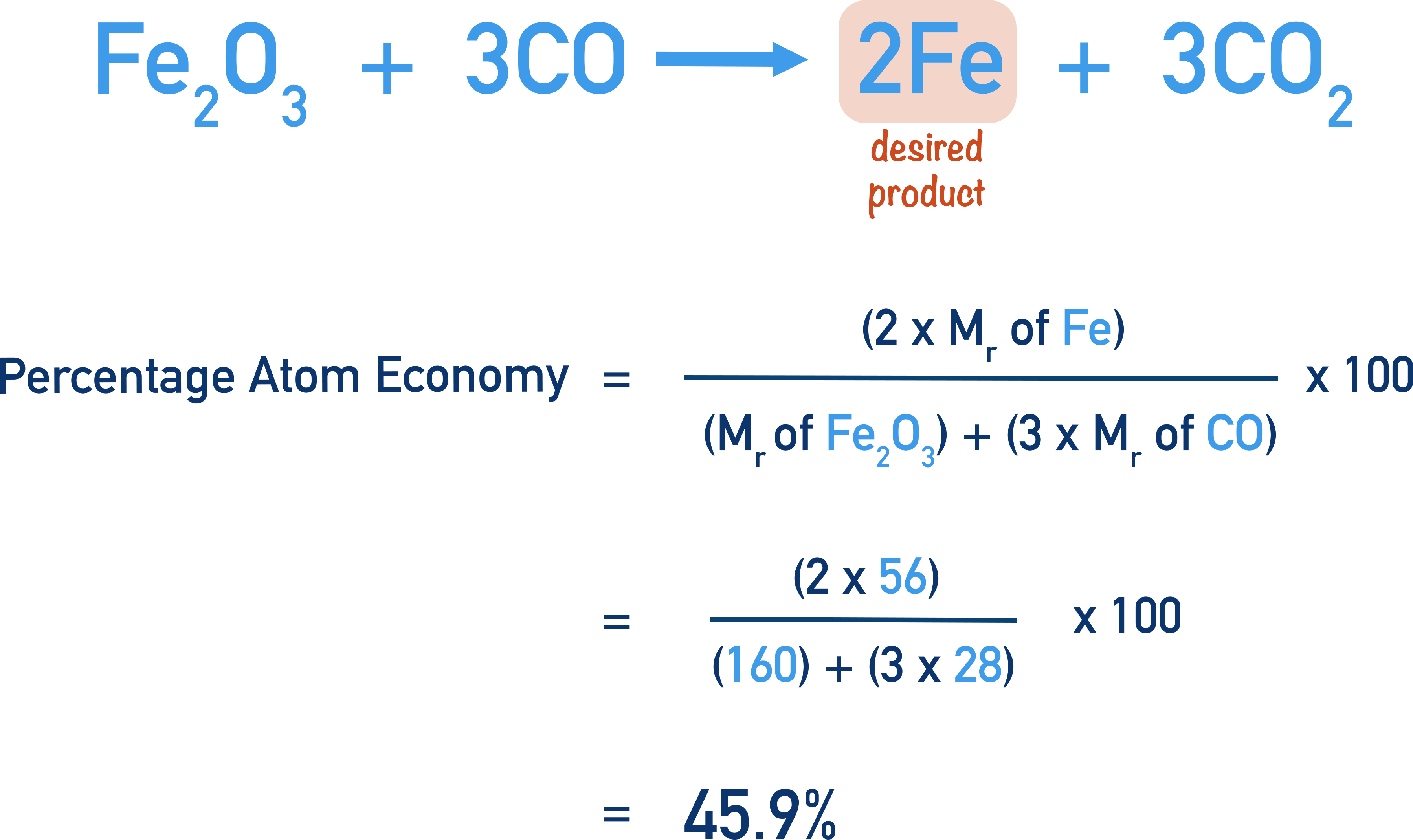Find the percentage atom economy for the production of ethanol (CH3CH2OH) from the reaction between ethene (CH2CH2) and water (H2O).## Difference Between Atom Economy and Percentage Yield

It’s important to understand that the percentage atom economy for a reaction is a theoretical value. There is no connection between the atom economy of a reaction and percentage yield.

Yield refers to how much product is obtained.

Percentage yield is experimental – it’s a comparison between how much product COULD be made in a reaction and the ACTUAL amount obtained. Percentage yields can be affected by practical methods and how a reaction is carried out.

Percentage atom economy has no connection to practical methods or experimental data. It’s always the same – the percentage atom economy for a particular reaction can never be changed.